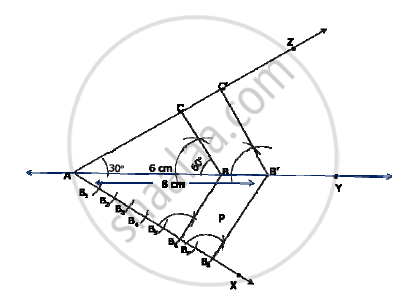# Construct a Δ ABC in which AB = 6 cm, ∠A = 30° and ∠B = 60°, Construct another ΔAB’C’ similar to ΔABC with base AB’ = 8 cm. - Mathematics

Construct a Δ ABC in which AB = 6 cm, ∠A = 30° and ∠B = 60°, Construct another ΔAB’C’ similar to ΔABC with base AB’ = 8 cm.

#### Solution

Construct the Δ ABC as per given measurements.

1) in the half plane if bar(AB) which does not contain C,

2)draw vec(AX) such that ∠BAX is an acute angle

3) With some appropriate radius and centre A, Draw an arc to intersect vec(AX) at B_1. Similarly, with center B_1 and same radius, drawn an arc to intersect vec(BX) at B2 such that B_1B_2=B_3B_4=B_4B_5=B_5B_6=B_6B_7=B_7B_8

4) Draw bar(B6B)

5) Through B8 draw a ray parallel to bar(B6B).to intersect bar(AY) at B'.

6) Through B' draw aray parallel to bar (BC) to intersect bar(AZ) at C' thus , ΔAB'C' is required triangleConcept: Division of a Line Segment
Is there an error in this question or solution?